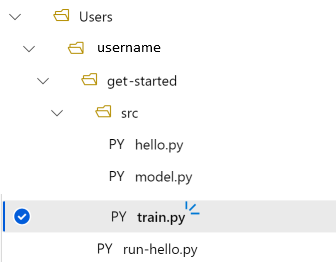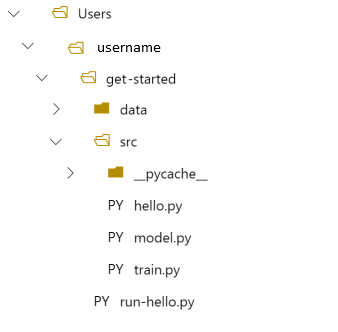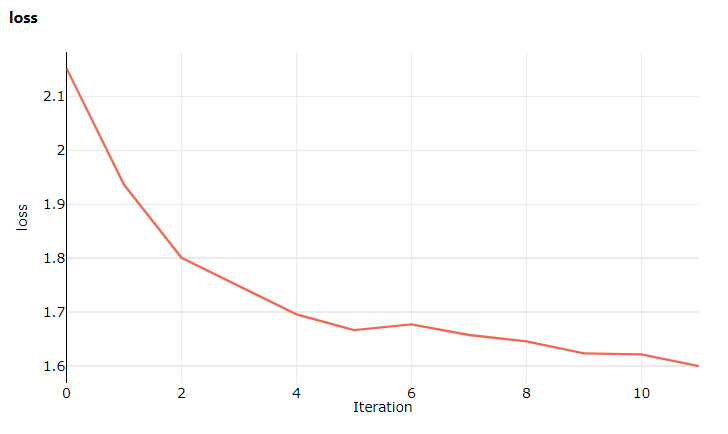# 教程：训练你的第一个机器学习模型（第 2 部分，共 3 部分）

• 创建训练脚本。
• 使用 Conda 定义 Azure 机器学习环境。
• 创建控制脚本。
• 了解 Azure 机器学习类（`Environment``Run``Metrics`）。
• 提交并运行训练脚本。
• 在云中查看代码输出。
• 将指标记录到 Azure 机器学习。
• 在云中查看指标。

## 创建训练脚本

1. 在 src 子文件夹中创建 model.py。 将以下代码复制到文件中：

``````import torch.nn as nn
import torch.nn.functional as F
class Net(nn.Module):
def __init__(self):
super(Net, self).__init__()
self.conv1 = nn.Conv2d(3, 6, 5)
self.pool = nn.MaxPool2d(2, 2)
self.conv2 = nn.Conv2d(6, 16, 5)
self.fc1 = nn.Linear(16 * 5 * 5, 120)
self.fc2 = nn.Linear(120, 84)
self.fc3 = nn.Linear(84, 10)
def forward(self, x):
x = self.pool(F.relu(self.conv1(x)))
x = self.pool(F.relu(self.conv2(x)))
x = x.view(-1, 16 * 5 * 5)
x = F.relu(self.fc1(x))
x = F.relu(self.fc2(x))
x = self.fc3(x)
return x
``````
2. 在工具栏中，选择“保存”以保存文件。 如果需要，请关闭选项卡。

3. 接下来，还是在 src 子文件夹中定义训练脚本。 此脚本通过使用 PyTorch `torchvision.dataset` API 来下载 CIFAR10 数据集，设置 model.py 中定义的网络，并通过使用标准 SGD 和互熵损失对该数据集进行两个时期的训练。

在 src 子文件夹中创建 train.py 脚本：

``````import torch
import torch.optim as optim
import torchvision
import torchvision.transforms as transforms

from model import Net

trainset = torchvision.datasets.CIFAR10(
root="../data",
train=True,
transform=torchvision.transforms.ToTensor(),
)
trainset, batch_size=4, shuffle=True, num_workers=2
)

if __name__ == "__main__":

# define convolutional network
net = Net()

# set up pytorch loss /  optimizer
criterion = torch.nn.CrossEntropyLoss()
optimizer = optim.SGD(net.parameters(), lr=0.001, momentum=0.9)

# train the network
for epoch in range(2):

running_loss = 0.0
for i, data in enumerate(trainloader, 0):
# unpack the data
inputs, labels = data

# forward + backward + optimize
outputs = net(inputs)
loss = criterion(outputs, labels)
loss.backward()
optimizer.step()

# print statistics
running_loss += loss.item()
if i % 2000 == 1999:
loss = running_loss / 2000
print(f"epoch={epoch + 1}, batch={i + 1:5}: loss {loss:.2f}")
running_loss = 0.0

print("Finished Training")
``````
4. 现在你拥有以下文件夹结构：## 本地测试## 创建控制脚本

``````# run-pytorch.py
from azureml.core import Workspace
from azureml.core import Experiment
from azureml.core import Environment
from azureml.core import ScriptRunConfig

if __name__ == "__main__":
ws = Workspace.from_config()
experiment = Experiment(workspace=ws, name='day1-experiment-train')
config = ScriptRunConfig(source_directory='./src',
script='train.py',
compute_target='cpu-cluster')

# use curated pytorch environment
env = ws.environments['AzureML-PyTorch-1.6-CPU']
config.run_config.environment = env

run = experiment.submit(config)

aml_url = run.get_portal_url()
print(aml_url)
``````

### 了解代码更改

`env = ...`

Azure 机器学习提供了环境概念来表示一个可重现的、进行了版本控制的 Python 环境，以便用来运行试验。 从本地 Conda 或 pip 环境创建一个环境很简单。

`config.run_config.environment = env`

## 将该运行提交到 Azure 机器学习

``````Downloading https://www.cs.toronto.edu/~kriz/cifar-10-python.tar.gz to ./data/cifar-10-python.tar.gz
...
epoch=1, batch= 2000: loss 2.19
epoch=1, batch= 4000: loss 1.82
epoch=1, batch= 6000: loss 1.66
epoch=1, batch= 8000: loss 1.58
epoch=1, batch=10000: loss 1.52
epoch=1, batch=12000: loss 1.47
epoch=2, batch= 2000: loss 1.39
epoch=2, batch= 4000: loss 1.38
epoch=2, batch= 6000: loss 1.37
epoch=2, batch= 8000: loss 1.33
epoch=2, batch=10000: loss 1.31
epoch=2, batch=12000: loss 1.27
Finished Training
``````

## 记录训练指标

### 修改 train.py 以包含日志记录

1. 修改 train.py 脚本，以包含另外两行代码：

``````import torch
import torch.optim as optim
import torchvision
import torchvision.transforms as transforms
from model import Net
from azureml.core import Run
# ADDITIONAL CODE: get run from the current context
run = Run.get_context()
trainset = torchvision.datasets.CIFAR10(
root='./data',
train=True,
transform=torchvision.transforms.ToTensor()
)
trainset,
batch_size=4,
shuffle=True,
num_workers=2
)
if __name__ == "__main__":
# define convolutional network
net = Net()
# set up pytorch loss /  optimizer
criterion = torch.nn.CrossEntropyLoss()
optimizer = optim.SGD(net.parameters(), lr=0.001, momentum=0.9)
# train the network
for epoch in range(2):
running_loss = 0.0
for i, data in enumerate(trainloader, 0):
# unpack the data
inputs, labels = data
# forward + backward + optimize
outputs = net(inputs)
loss = criterion(outputs, labels)
loss.backward()
optimizer.step()
# print statistics
running_loss += loss.item()
if i % 2000 == 1999:
loss = running_loss / 2000
# ADDITIONAL CODE: log loss metric to AML
run.log('loss', loss)
print(f'epoch={epoch + 1}, batch={i + 1:5}: loss {loss:.2f}')
running_loss = 0.0
print('Finished Training')
``````
2. 保存此文件，然后根据需要关闭该选项卡。

#### 了解这两行额外添加的代码

``````# ADDITIONAL CODE: get run from the current context
run = Run.get_context()

...
# ADDITIONAL CODE: log loss metric to AML
run.log('loss', loss)
``````

Azure 机器学习中的指标具有以下特点：

• 按试验和运行进行组织，因此可以轻松地跟踪和比较指标。
• 配备了一个 UI，使你能够在工作室中可视化训练性能。
• 设计用于进行扩展，因此即使在运行数百个试验的情况下，你也始终有这些优势。

### 将该运行提交到 Azure 机器学习